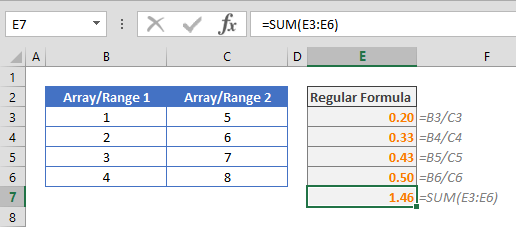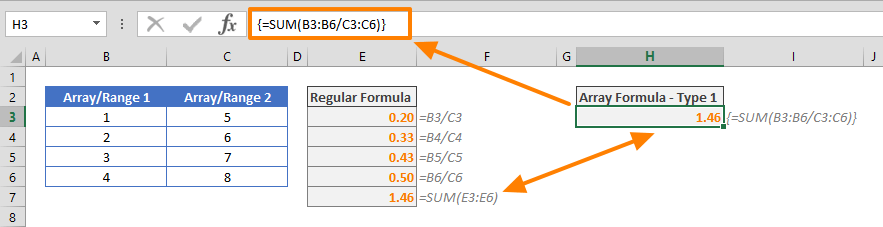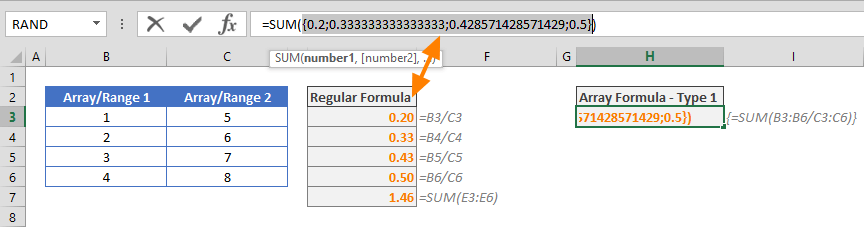Control Shift Enter Excel shortcut, as known as CSE, is a key combination you can use to apply array functions.CSE abbreviation is also used for array formulas that are entered using the Control + Shift + Enter key combination when entering a formula. Array formulas are slightly different than regular formulas that are entered by only pressing the Enter key. In this guide, we're going to show you how to use the Control Shift Enter Excel shortcut (CSE).

## Array formulas (CSE)

Array formulas are an Excel feature that allow executing complex operations regular formulas can't. When a formula is defined as a regular formula, the functions and operations evaluate ranges and arrays as is. Let's see the differences between these two approached on an example.

### Multiple actions in a single cell

In our example, there are two ranges with numbers. We want to sum up values after dividing each value in the first array by the corresponding value in the second. With the regular formula approach, you need to divide each cell in the next column and sum the values from the next column.This approach needs at least two type of formulas for dividing and summing. Also, you need to divide through the helper column.

Using Control Shift Enter Excel shortcut, you can do this operation in one cell! Instead of using the helper range reference, E3:E6, in the SUM function, you can use the references of ranges in the function and evaluate each operation together.As you can see in the example above, the formula in cell H3 returns the same value used in the regular method. CSE in Excel removes the need of a helper column. Let's see how it works.

{=SUM(B3:B6/C3:C6)}

First of all, you may have noticed the curly brackets surrounding the formula. These brackets are indicators of an array formula. Do not put curly brackets yourself - Excel places them automatically if you press the CSE combination to submit the formula.

To evaluate a formula like this,

1. select the cell that contains the array formula
2. in the formula bar, select the "B3:B6/C3:C6" part
3. and press the F9 keyExcel generates an array from the selected range, dividing the values. The beauty of array formulas is that Excel handles them as if they are a part of the formulas instead of requiring a helper column.

### Populating multiple cells

If you want to populate an array of values into cells, you can use array formulas as well. The first example was using SUM function to sum the values of an array. The SUM function returns only a single value. Let's now take a look at how the formula would work if the SUM function wasn't used. If you exclude SUM function, you will get something like the following:

{=B3:B6/C3:C6}

If you select a single cell and enter the formula above, you will get 0.20 which is equal to 1/5. This may seem off, but if you select a range of 4 rows and 1 column and enter the formula as an array function, you will see all cells are populated correctly.There is a restriction for when you populate a range with an array formula: When you want to update or delete the formula, you need to select the entire range every time you need to modify it. Otherwise, Excel will give you a warning.## Evaluating arrays without CSE

Array formulas are not the only solution to simplifying complex operations. Especially, this example assumes that you want to return a single value, and Excel's SUMPRODUCT function is a powerful formula that can evaluate arrays as is. Here is a formula that uses our previous example:

=SUMPRODUCT(B3:B6/C3:C6)## Functions that return arrays

Some Excel functions can return arrays without using them in an array formula. Below are some common examples:

• TRANSPOSE
• OFFSET
• INDEX
• LINEST
• LOGEST

Please note that the TRANSPOSE function can only be used with the CSE combination. Otherwise, you will get a #VALUE! error. The remaining functions return the first values in the return array.